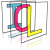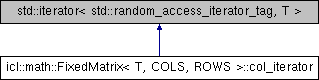Image Component Library (ICL)
icl::math::FixedMatrix< T, COLS, ROWS >::col_iterator Struct Reference

internal struct for row-wise iteration with stride=COLS More...

`#include <FixedMatrix.h>`

Inheritance diagram for icl::math::FixedMatrix< T, COLS, ROWS >::col_iterator:## Public Types

typedef unsigned int difference_type
just for compatibility with STL More...

## Public Member Functions

col_iterator (T *col_begin)
Constructor. More...

col_iteratoroperator++ ()
prefix increment operator More...

const col_iteratoroperator++ () const
prefix increment operator (const) More...

col_iterator operator++ (int)
postfix increment operator More...

const col_iterator operator++ (int) const
postfix increment operator (const) More...

col_iteratoroperator-- ()
prefix decrement operator More...

const col_iteratoroperator-- () const
prefix decrement operator (const) More...

col_iterator operator-- (int)
postfix decrement operator More...

const col_iterator operator-- (int) const
postfix decrement operator (const) More...

col_iteratoroperator+= (difference_type n)
jump next n elements (inplace) More...

const col_iteratoroperator+= (difference_type n) const
jump next n elements (inplace) (const) More...

col_iteratoroperator-= (difference_type n)
jump backward next n elements (inplace) More...

const col_iteratoroperator-= (difference_type n) const
jump backward next n elements (inplace) (const) More...

col_iterator operator+ (difference_type n)
jump next n elements More...

const col_iterator operator+ (difference_type n) const
jump next n elements (const) More...

col_iterator operator- (difference_type n)
jump backward next n elements More...

const col_iterator operator- (difference_type n) const
jump backward next n elements (const) More...

difference_type operator- (const col_iterator &i) const
steps between two iterators ... (todo: check!) More...

T & operator * ()
Dereference operator. More...

const T & operator * () const
const Dereference operator More...

bool operator== (const col_iterator &i) const
comparison operator == More...

bool operator!= (const col_iterator &i) const
comparison operator != More...

bool operator< (const col_iterator &i) const
comparison operator < More...

bool operator<= (const col_iterator &i) const
comparison operator <= More...

bool operator>= (const col_iterator &i) const
comparison operator >= More...

bool operator> (const col_iterator &i) const
comparison operator > More...

## Public Attributes

T * p
wrapped data pointer (held shallowly) More...

## Static Public Attributes

static const unsigned int STRIDE = COLS
the stride is equal to parent Matrix' classes COLS template parameter More...

## Detailed Description

### template<class T, unsigned int COLS, unsigned int ROWS> struct icl::math::FixedMatrix< T, COLS, ROWS >::col_iterator

internal struct for row-wise iteration with stride=COLS

## ◆ difference_type

template<class T, unsigned int COLS, unsigned int ROWS>
 typedef unsigned int icl::math::FixedMatrix< T, COLS, ROWS >::col_iterator::difference_type

just for compatibility with STL

## ◆ col_iterator()

template<class T, unsigned int COLS, unsigned int ROWS>
 icl::math::FixedMatrix< T, COLS, ROWS >::col_iterator::col_iterator ( T * col_begin )
inline

Constructor.

## ◆ operator *() [1/2]

template<class T, unsigned int COLS, unsigned int ROWS>
 T& icl::math::FixedMatrix< T, COLS, ROWS >::col_iterator::operator * ( )
inline

Dereference operator.

## ◆ operator *() [2/2]

template<class T, unsigned int COLS, unsigned int ROWS>
 const T& icl::math::FixedMatrix< T, COLS, ROWS >::col_iterator::operator * ( ) const
inline

const Dereference operator

## ◆ operator!=()

template<class T, unsigned int COLS, unsigned int ROWS>
 bool icl::math::FixedMatrix< T, COLS, ROWS >::col_iterator::operator!= ( const col_iterator & i ) const
inline

comparison operator !=

## ◆ operator+() [1/2]

template<class T, unsigned int COLS, unsigned int ROWS>
 col_iterator icl::math::FixedMatrix< T, COLS, ROWS >::col_iterator::operator+ ( difference_type n )
inline

jump next n elements

## ◆ operator+() [2/2]

template<class T, unsigned int COLS, unsigned int ROWS>
 const col_iterator icl::math::FixedMatrix< T, COLS, ROWS >::col_iterator::operator+ ( difference_type n ) const
inline

jump next n elements (const)

## ◆ operator++() [1/4]

template<class T, unsigned int COLS, unsigned int ROWS>
 col_iterator& icl::math::FixedMatrix< T, COLS, ROWS >::col_iterator::operator++ ( )
inline

prefix increment operator

## ◆ operator++() [2/4]

template<class T, unsigned int COLS, unsigned int ROWS>
 const col_iterator& icl::math::FixedMatrix< T, COLS, ROWS >::col_iterator::operator++ ( ) const
inline

prefix increment operator (const)

## ◆ operator++() [3/4]

template<class T, unsigned int COLS, unsigned int ROWS>
 col_iterator icl::math::FixedMatrix< T, COLS, ROWS >::col_iterator::operator++ ( int )
inline

postfix increment operator

## ◆ operator++() [4/4]

template<class T, unsigned int COLS, unsigned int ROWS>
 const col_iterator icl::math::FixedMatrix< T, COLS, ROWS >::col_iterator::operator++ ( int ) const
inline

postfix increment operator (const)

## ◆ operator+=() [1/2]

template<class T, unsigned int COLS, unsigned int ROWS>
 col_iterator& icl::math::FixedMatrix< T, COLS, ROWS >::col_iterator::operator+= ( difference_type n )
inline

jump next n elements (inplace)

## ◆ operator+=() [2/2]

template<class T, unsigned int COLS, unsigned int ROWS>
 const col_iterator& icl::math::FixedMatrix< T, COLS, ROWS >::col_iterator::operator+= ( difference_type n ) const
inline

jump next n elements (inplace) (const)

## ◆ operator-() [1/3]

template<class T, unsigned int COLS, unsigned int ROWS>
 col_iterator icl::math::FixedMatrix< T, COLS, ROWS >::col_iterator::operator- ( difference_type n )
inline

jump backward next n elements

## ◆ operator-() [2/3]

template<class T, unsigned int COLS, unsigned int ROWS>
 const col_iterator icl::math::FixedMatrix< T, COLS, ROWS >::col_iterator::operator- ( difference_type n ) const
inline

jump backward next n elements (const)

## ◆ operator-() [3/3]

template<class T, unsigned int COLS, unsigned int ROWS>
 difference_type icl::math::FixedMatrix< T, COLS, ROWS >::col_iterator::operator- ( const col_iterator & i ) const
inline

steps between two iterators ... (todo: check!)

## ◆ operator--() [1/4]

template<class T, unsigned int COLS, unsigned int ROWS>
 col_iterator& icl::math::FixedMatrix< T, COLS, ROWS >::col_iterator::operator-- ( )
inline

prefix decrement operator

## ◆ operator--() [2/4]

template<class T, unsigned int COLS, unsigned int ROWS>
 const col_iterator& icl::math::FixedMatrix< T, COLS, ROWS >::col_iterator::operator-- ( ) const
inline

prefix decrement operator (const)

## ◆ operator--() [3/4]

template<class T, unsigned int COLS, unsigned int ROWS>
 col_iterator icl::math::FixedMatrix< T, COLS, ROWS >::col_iterator::operator-- ( int )
inline

postfix decrement operator

## ◆ operator--() [4/4]

template<class T, unsigned int COLS, unsigned int ROWS>
 const col_iterator icl::math::FixedMatrix< T, COLS, ROWS >::col_iterator::operator-- ( int ) const
inline

postfix decrement operator (const)

## ◆ operator-=() [1/2]

template<class T, unsigned int COLS, unsigned int ROWS>
 col_iterator& icl::math::FixedMatrix< T, COLS, ROWS >::col_iterator::operator-= ( difference_type n )
inline

jump backward next n elements (inplace)

## ◆ operator-=() [2/2]

template<class T, unsigned int COLS, unsigned int ROWS>
 const col_iterator& icl::math::FixedMatrix< T, COLS, ROWS >::col_iterator::operator-= ( difference_type n ) const
inline

jump backward next n elements (inplace) (const)

## ◆ operator<()

template<class T, unsigned int COLS, unsigned int ROWS>
 bool icl::math::FixedMatrix< T, COLS, ROWS >::col_iterator::operator< ( const col_iterator & i ) const
inline

comparison operator <

## ◆ operator<=()

template<class T, unsigned int COLS, unsigned int ROWS>
 bool icl::math::FixedMatrix< T, COLS, ROWS >::col_iterator::operator<= ( const col_iterator & i ) const
inline

comparison operator <=

## ◆ operator==()

template<class T, unsigned int COLS, unsigned int ROWS>
 bool icl::math::FixedMatrix< T, COLS, ROWS >::col_iterator::operator== ( const col_iterator & i ) const
inline

comparison operator ==

## ◆ operator>()

template<class T, unsigned int COLS, unsigned int ROWS>
 bool icl::math::FixedMatrix< T, COLS, ROWS >::col_iterator::operator> ( const col_iterator & i ) const
inline

comparison operator >

## ◆ operator>=()

template<class T, unsigned int COLS, unsigned int ROWS>
 bool icl::math::FixedMatrix< T, COLS, ROWS >::col_iterator::operator>= ( const col_iterator & i ) const
inline

comparison operator >=

## ◆ p

template<class T, unsigned int COLS, unsigned int ROWS>
 T* icl::math::FixedMatrix< T, COLS, ROWS >::col_iterator::p
mutable

wrapped data pointer (held shallowly)

## ◆ STRIDE

template<class T, unsigned int COLS, unsigned int ROWS>
 const unsigned int icl::math::FixedMatrix< T, COLS, ROWS >::col_iterator::STRIDE = COLS
static

the stride is equal to parent Matrix' classes COLS template parameter

The documentation for this struct was generated from the following file: